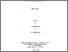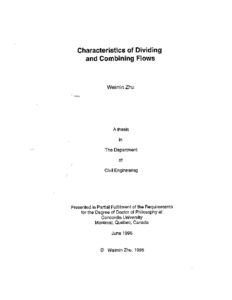Title:

# Characteristics of dividing and combining flows

Zhu, Weimin (1995) Characteristics of dividing and combining flows. PhD thesis, Concordia University.Preview
Text (application/pdf)
NN05069.pdf
3MB

## Abstract

In the present thesis, some aspects of dividing and combining flows are studied. The first part of the study considers the discharge characteristics of flow past a two-dimensional lateral slot located in a rectangular conduit. Existing theoretical solutions to this problem are based on free streamline theory. The test results provide data to verify the predictions of the theoretical model related to the dependence of the slot discharge coefficient on discharge ratios and the ratio of the kinetic energy to the total energy of the flow approaching the slot. The test results validate the model predictions. A theoretical expression for the outflow through a rectangular lateral weir located in a circular open channel is derived. The theoretical weir discharge coefficient is expressed as a function of the parameters relating the geometry of the weir and the channel, and a velocity parameter that is a function of the dimensionless flow depth, the weir sill height and the approach Froude number. Experimental results are presented to verify the proposed theoretical model. The characteristics of dividing flows in closed rectangular conduits are studied in the next section. The contraction coefficient and the loss coefficients of branching flows are determined using results of an existing model dealing with a two-dimensional lateral outlet fitted with an external barrier. For dividing closed conduit flows, two different procedures are used to obtain the variation of an energy loss coefficient and the contraction coefficient with the discharge ratio. Detailed velocity and pressure distribution profiles are presented to describe the flow processes. In the final part of the thesis, the characteristics of combining flows past 90\$\sp\circ\$ junctions of a rectangular closed conduit are presented. Detailed pressure and velocity distribution data are obtained to understand the flow processes. Both the mean and fluctuating components of the velocity field are determined in the two flow sections immediately downstream of the junction. Simple empirical models are developed to determine the transfer of momentum and the contraction coefficient. Experimental data are used to verify the predicted results of the proposed models

Divisions: Concordia University > Gina Cody School of Engineering and Computer Science > Building, Civil and Environmental Engineering Thesis (PhD) Zhu, Weimin xviii, 162 leaves : ill. ; 29 cm. Concordia University Ph. D. Building, Civil and Environmental Engineering 1995 Ramamurthy, A. S 106 Concordia University Library 27 Aug 2009 17:09 18 Jan 2018 17:12 http://clues.concordia.ca/search/c?SEARC...
All items in Spectrum are protected by copyright, with all rights reserved. The use of items is governed by Spectrum's terms of access.

Repository Staff Only: item control pageResearch related to the current document (at the CORE website)
Back to top